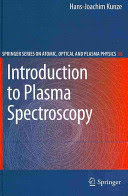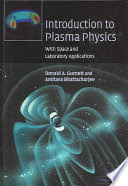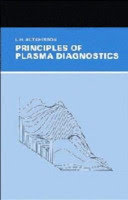### Fundamentals of Plasma Physics

#### Learning Outcomes

1. Define plasma state and characteristic plasma parameters.
2. Explain plasma and cyclotron frequency.
3. Describe plasma system with magnetohydrodynamics.
4. Describe collisional processes in plasma.
5. Describe waves in plasma.
6. Describe plasma types and their use.
7. Describe plasma system instabilities.

#### Forms of Teaching

Lectures

2 cycles of lectures: 7 weeks and 6 weeks

Seminars and workshops

non mandatory seminar

Exercises

problems and exercises

Partial e-learning

Continuous Assessment Exam
Class participation 0 % 5 % 0 % 5 %
Seminar/Project 0 % 25 % 0 % 25 %
Mid Term Exam: Written 0 % 35 % 0 %
Final Exam: Written 0 % 35 %
Exam: Written 0 % 70 %

#### Week by Week Schedule

1. Definition of plasma state; Ionization and recombination processes; Degree of ionization; Pressure and the mean kinetic energy of a gas particle; Equation of state; Degrees of freedom of the molecule; Thermal capacities of gases.
2. Descriptions of plasma systems; Characteristic plasma parameters; Bohr's postulates; Bohr's model of the hydrogen atom; Quantization of energy; Maxwell distribution of particle velocities; Equipartition of energy and Maxwell-Boltzmann distribution.
3. Collective interaction; Quasineutrality; Plasma and cyclotron frequency; Thermodynamic equilibrium.
4. Electron plasma frequency.
5. Debye length, Debye shielding; Classical and quantum regime; Electrostatic plasma waves, Landau damping.
6. Collision frequency; Collisions between charged and neutral particles; Collisions between charged particles; Nuclear fusion; Photoionization and excitation; Electron impact ionization; Collisions with surfaces.
7. Plasma - fluid; Equation of motion; Equation of continuity; Equation of state; Maxwell equations in plasma.
8. Midterm exam.
9. Instabilities; Plasma instabilities; MHD instabilities.
10. Plasma diagnostics: determination of temperature.
11. Plasma diagnostics: determination of density.
12. Application of plasma: artificially produced plasma.
13. Application of plasma: plasma on Earth.
14. Application of plasma: plasma in space.
15. Final exam.

#### Study Programmes

Computing (study)
Elective Courses (5. semester)
Electrical Engineering and Information Technology (study)
Elective Courses (5. semester)

#### Literature

Sanda Pleslić (2018.), Osnove fizike plazme (elektronička skripta) 2010.-2018.,H.-J. Kunze (2009.), Introduction to Plasma Spetroscopy, SpringerD. A. Gurnett, A. Bhattacharjee (2005.), Introduction to Plasma Physics, Cambridge University Press
A. W. DeSilva (1991.), Plasma Diagnostics, University of MarylandI. H. Hutchinson (1987.), Principles of Plasma Diagnostics, Cambrigdge University Press
P. M. Belan (2006.), Fundamentals of Plasma Physics, Cambridge University Press

#### General

ID 183406
Winter semester
5 ECTS
L3 English Level
L1 e-Learning
45 Lectures
15 Exercises
0 Laboratory exercises
0 Project laboratory